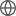Old Web
English
# Radio and Far-IR Emission Associated with a Massive Star-forming Galaxy Candidate at z$\simeq$6.8: A Radio-Loud AGN in the Reionization Era?
We report the identification of radio (1.4 and 3 GHz) and mid-infrared, far-infrared, and sub-mm (24-850$\mu$m) emission at the position of one of 41 UV-bright ($\mathrm{M_{UV}^{}}\lesssim-21.25$) $z\simeq6.6-6.9$ Lyman-break galaxy candidates in the 1.5 deg$^2$ COSMOS field. This source, COS-87259, exhibits a sharp flux discontinuity (factor $>$3) between two narrow/intermediate bands at 9450 $\mathring{A}$ and 9700 $\mathring{A}$ and is undetected in all nine bands blueward of 9600 $\mathring{A}$, as expected from a Lyman-alpha break at $z\simeq6.8$. The full multi-wavelength (X-ray through radio) data of COS-87529 can be self-consistently explained by a very massive (M$_{\ast}=10^{10.8}$ M$_{\odot}$) and extremely red (rest-UV slope $\beta=-0.59$) $z\simeq6.8$ galaxy with hyperluminous infrared emission (L$_{\mathrm{IR}}=10^{13.6}$ L$_{\odot}$) powered by both an intense burst of highly-obscured star formation (SFR$\approx$1800 M$_{\odot}$ yr$^{-1}$) and an obscured ($\tau_{\mathrm{9.7\mu m}}=7.7\pm2.5$) radio-loud (L$_{\mathrm{1.4\ GHz}}\sim10^{25.5}$ W Hz$^{-1}$) AGN. The radio emission is compact (1.04$\pm$0.12 arcsec) and exhibits an ultra-steep spectrum between 1.4-3 GHz ($\alpha=-2.06^{+0.27}_{-0.25}$) with evidence of spectral flattening at lower frequencies, consistent with known $z>4$ radio galaxies. We also demonstrate that COS-87259 may reside in a significant (11$\times$) galaxy overdensity at $z\simeq6.6-6.9$, as common for systems hosting radio-loud AGN. Nonetheless, a spectroscopic redshift will ultimately be required to establish the true nature of COS-87259 as we cannot yet completely rule out low-redshift solutions. If confirmed to lie at $z\simeq6.8$, the properties of COS-87259 would be consistent with a picture wherein AGN and highly-obscured star formation activity are fairly common among very massive (M$_{\ast}>10^{10}$ M$_{\odot}$) reionization-era galaxies.